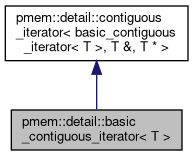PMDK C++ bindings  1.13.0-git85.g2ab46040 This is the C++ bindings documentation for PMDK's libpmemobj.
pmem::detail::basic_contiguous_iterator< T > Struct Template Reference

Default non-const iterator which adds element to a transaction on every access. More...

#include <libpmemobj++/container/detail/contiguous_iterator.hpp>

Inheritance diagram for pmem::detail::basic_contiguous_iterator< T >:[legend]

Public Types

using iterator_category = std::random_access_iterator_tag

using value_type = T

using difference_type = std::ptrdiff_t

using reference = T &

using pointer = T *

using base_type = contiguous_iterator< basic_contiguous_iterator< T >, reference, pointer >

Public Member Functions

basic_contiguous_iterator (pointer ptr=nullptr)
Constructor taking pointer and snapshotting function as arguments.

operator const T * () const
Conversion operator to const T*.

reference operator* () const
Dereference operator which adds dereferenced element to a transaction.

pointer operator-> () const
Arrow operator which adds underlying element to a transactions.

reference operator[] (std::ptrdiff_t n)
Element access operator. More...

basic_contiguous_iterator< T > & operator++ ()
Prefix increment operator.

basic_contiguous_iterator< T > operator++ (int)
Postfix increment operator.

basic_contiguous_iterator< T > & operator-- ()
Prefix decrement operator.

basic_contiguous_iterator< T > operator-- (int)
Postfix decrement operator.

basic_contiguous_iterator< T > & operator+= (std::ptrdiff_t n)

basic_contiguous_iterator< T > & operator-= (std::ptrdiff_t n)
Subtraction assignment operator.

basic_contiguous_iterator< T > operator+ (std::ptrdiff_t n) const

basic_contiguous_iterator< T > operator- (std::ptrdiff_t n) const
Subtraction operator overload for integral type.

T * get_ptr () const

Protected Member Functions

void change_by (std::ptrdiff_t n)
Function for changing underlying pointer. More...

T * ptr

Friends

void swap (basic_contiguous_iterator &lhs, basic_contiguous_iterator &rhs)
Non-member swap function.

Detailed Description

template<typename T> struct pmem::detail::basic_contiguous_iterator< T >

Default non-const iterator which adds element to a transaction on every access.

◆ change_by()

 void pmem::detail::contiguous_iterator< basic_contiguous_iterator< T > , T & , T * >::change_by ( std::ptrdiff_t n )
inlineprotectedinherited

Function for changing underlying pointer.

This is where static polymorphism is used. Derived classes can override this method and snapshot data if necessary.

◆ operator[]()

template<typename T >
 reference pmem::detail::basic_contiguous_iterator< T >::operator[] ( std::ptrdiff_t n )
inline

Element access operator.

Adds range containing specified element to a transaction.

The documentation for this struct was generated from the following file: